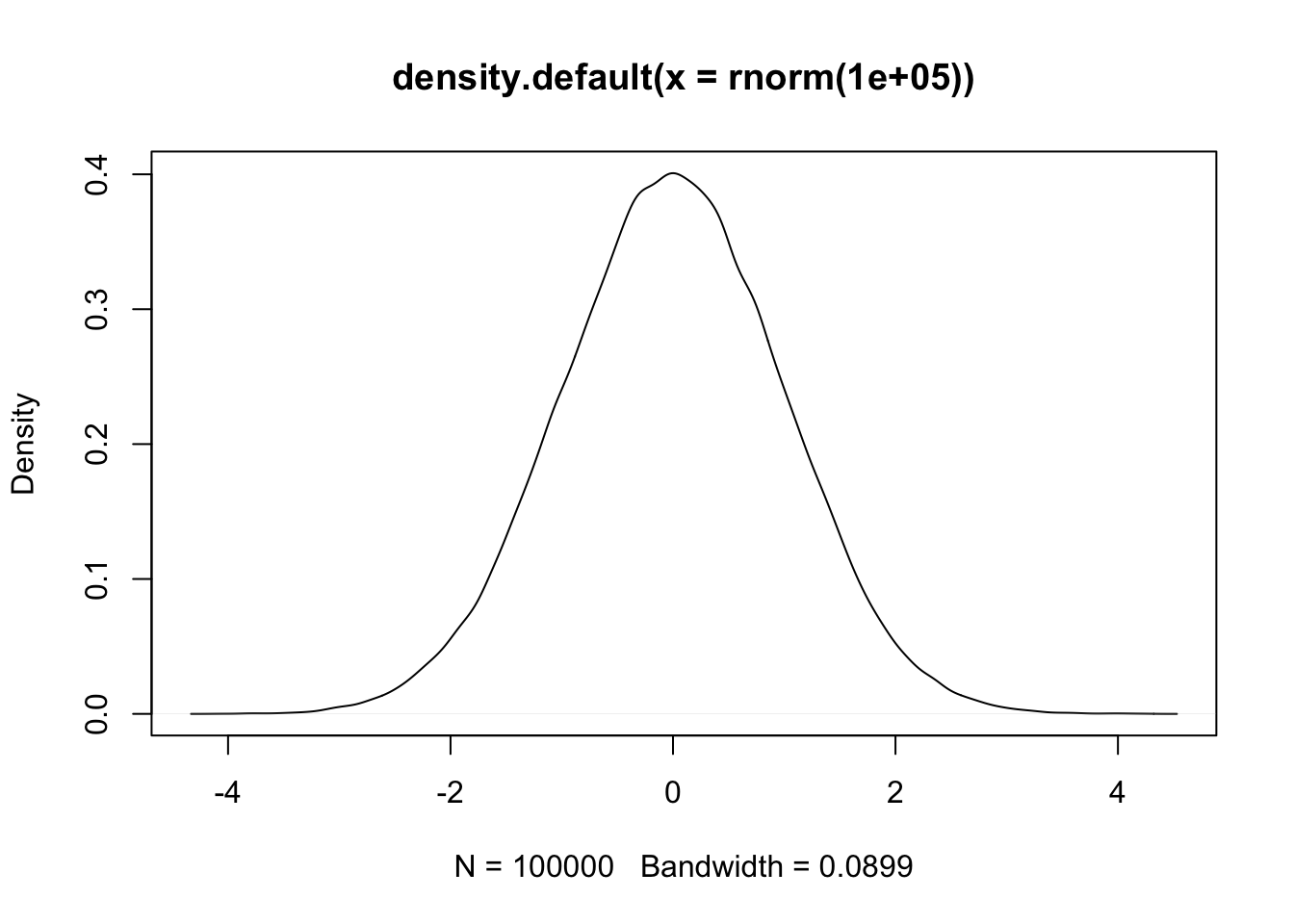This document provides answers to the questions in HW0. Feel free to peruse it or use it as a reference.

# 1 Basic Statistics

You should feel very comfortable with everything in this section.

## 1.1 Probability Statements

1. Interpret the statement “this is a fair coin.”

Any given toss of the coin is as likely to be heads as tails.

1. Out of 25 coin tosses, how many do you anticipate will be heads?

12.5

1. What do we conclude if all 25 are heads?

It is extremely unlikely the coin is fair.

1. I have a new coin. Will you make a bet with me about the next flip?

Absolutely not. But I might be willing to bet with someone you don’t know about its flip.

1. Find the flaw in the following statement “The probability of a nuclear accident resulting in the death of at least one US resident in the next 5 years is either 0 or 1 but we won’t know for 5 years”.

Most prominently, it begs the question, “what is the probability that probability is 1?”. More importantly, it is an utterly unhelpful prediction.

1. P[x>6] = 0.4; P[x>10] = 0 = P[x<0]. Interpet in words.

There is some variable X taking values between 0 and 10. 40% of the time it is greater than 6.

1. If there is a 40% chance something happens, what are its odds?

The odds are 2 to 3.

## 1.2 Expectations

1. What is the expected value of the sum of the 25 coin tosses above?

12.5

1. Question 6 above gives some information about a variable x. What range of possible values could the expectation of X take?

E[x] depends on the (mostly unknown) distribution. The max possible value would happen when the distribution is as far right as possible. In this case, that happens when P[x=6]=0.6 and P[x=10]=0.4, giving a max expectation of 7.6. The min is when the distribution is as far left as possible, at P[x=0]=0.6 and P[x=6+epsilon]=0.4, for a minimum expectation of 2.4+epsilon.

1. Interpret E[Y] = 0. E[Y|x=5] = 0. E[Y|Y=0] = ?

E[Y] = 0 says that the mean of Y is 0. E[Y|x=5] = 0 tells us that when x=5, the mean of Y is 0. E[Y|Y=0] is 0.

## 1.3 Variance

1. What is the variance of the sum of coin tosses above?

This is the variance of a binomial, which is np(1-p), or in this case: 25*0.25 = 6.25.

1. What is the range of possible variances of the variable X in question 6?

The variance is at a max when the distribution is as spread as possible. That would be when P[x=0]=0.6 and P[x=10]=0.4, for a variance of 24. The min variance is when the distribution is as narrow as possible, when P[x=6]=0.6 and P[x=6+epsilon]=0.4 for a variance of 0.24*epsilon^2. The range of variances possible is (0,24].

## 1.4 Density functions:

1. Interpret “the CDF of 2 is 0.6” and “the pdf of 2 is 0.6”. How do they differ?

The CDF of 2 is 0.6 means that 60% of values (of this unnamed random variable) are 2 or below. The pdf of 2 is 0.6 means that the density (of this unnamed RV) at 2 is 0.6, which is borderline uninterpretable by itself. The two are closely related in that the CDF is the running integral of the density, while the density is the slope of the CDF. Critically, “the pdf of 2 is 0.6” does not mean P[x=2]=0.6. Though “the cdf of 2 is 0.6” does mean P[x<=2] = 0.6.

# 2 Distributions

This section asks for details, but really you should just have some sense of these things, and know how to look up the true answers.

## 2.1 Gaussian/Normal

1. Draw a gaussian distribution.

I can’t easily draw this for you, so I’ll plot it in R. You certainly did not need to do that.

``plot(density(rnorm(100000)))``1. What is the mean of the gaussian distribution “N(0,1)”? Median? Mode? Variance? Standard Deviation? Kurtosis? Skew? 95% predictive interval?

Mean = 0. Median = 0. Mode = 0. Variance = 1, SD = 1. Kurtosis = 3 [excess kurtosis = 0]. Skew = 0. 95% predictive interval = (-1.96,1.96) [I would have been happy with (-2,2)].

## 2.2 t

1. Draw a t distribution on top of your gaussian distribution.

Same again

``````plot(density(rnorm(100000)))
lines(density(rt(100000,5)),col=2)``````